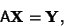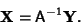## System of Equations

Let a linear system of equations be denoted(1)

whereis a Matrix and X and Y are Vectors. As shown by Cramer's Rule, there is a unique solution ifhas a Matrix Inverse. In this case,(2)

If, then the solution is. Ifhas no Matrix Inverse, then the solution Subspace is either a Line or the Empty Set. If two equations are multiples of each other, solutions are of the form(3)

fora Real Number.